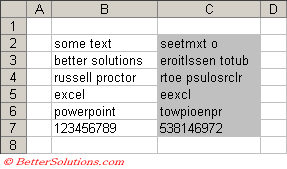# SCRAMBLE

Returns the cell contents with all the characters in a random order.

### Remarks

* For instructions on how to add a function to a workbook refer to the page under Inserting Functions
* The equivalent JavaScript function is SCRAMBLE

`'sCellContents - The number or text you want to scramble.Public Function SCRAMBLE(sCellContents As String) As String Dim itextlength As Integer Dim ichar As Integer Dim irandomposition As Integer Dim scharacter As String * 1     itextlength = Len(sCellContents)     For ichar = 1 To itextlength         scharacter = VBA.Mid(sCellContents, ichar, 1)         irandomposition = VBA.Int((itextlength - 1 + 1) * VBA.Rnd + 1)         Mid(sCellContents, ichar, 1) = VBA.Mid(sCellContents, irandomposition, 1)         Mid(sCellContents, irandomposition, 1) = scharacter     Next ichar         SCRAMBLE = sCellContents End Function `

You can use the RANDOMNUMBER function to return a random number between two bounds.
This user defined function is called "RANDOM" in the ASAP add-in.